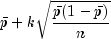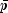JMSLTM Numerical Library 7.2.0
com.imsl.chart.qc

Class PChart

• All Implemented Interfaces:
Serializable, Cloneable

public class PChart
extends ShewhartControlChart
PChart is a p-chart for monitoring the defect rate when defects are not rare.

The defect rate is the number of defects found divided by the number of samples inspected. The number of defects are not assumed to be rare. Control limits are computed using the binomial distribution. If defects are rare, use UChart instead. The control limits are atwhereis the mean defect rate, n is the sample size, and k is the value of the "ControlLimit" attribute for the line. By default, the chart contains an upper control limit line with k=3, a lower control limit line with k=-3, and a central line equal to. Additional control limits can be added. The method addWeco adds control limits with k = -2, -1, 1, 2. The true fraction conforming p can be used by setting the attribute "Center" to p. The lower control limit is forced to have a minimum value of zero. The upper control limit is forced to have a maximum value of one.

Example, NIST Engineering Statistics Handbook: Proportions Control Charts, Serialized Form
• Constructor Detail

• PChart

public PChart(AxisXY axis,
int[] sampleSize,
double[] defectRate)
Creates a p-Chart given the defect rates for a series of samples with varying sample sizes.
Parameters:
axis - the AxisXY parent of this node
sampleSize - is an array containing the number of observations in each sample. It must be at least one.
defectRate - is an array containing defect rates of the samples. The defect rates must all be in the range [0,1]. The lengths of the arrays sampleSize and defectRate must be equal.
• PChart

public PChart(AxisXY axis,
int[] sampleSize,
int[] numberDefects)
Creates a p-Chart given the number of defects for a series of samples with varying sample sizes.
Parameters:
axis - the AxisXY parent of this node
sampleSize - is an array containing the number of observations in each sample. It must be at least one.
numberDefects - is an array of arrays containing the number of defects in each of a series of samples. The number of defects should not be less than zero.
• PChart

public PChart(AxisXY axis,
int sampleSize,
double[] defectRate)
Creates a p-Chart given the defect rates for a series of samples with equal sample sizes.
Parameters:
axis - the AxisXY parent of this node
sampleSize - is the number of observations in each sample. It must be at least one.
defectRate - is an array containing defect rates of the samples. The defect rates must all be in the range [0,1].
• PChart

public PChart(AxisXY axis,
int sampleSize,
int[] numberDefects)
Creates a p-Chart given the number of defects for a series of samples with equal sample sizes.
Parameters:
axis - the AxisXY parent of this node
sampleSize - is the number of observations in each sample. Each sample must contain at least one observation.
numberDefects - is an array containing the number of defects in each of a series of samples. The number of defects should not be less than zero.
• Method Detail

• prePaint

public void prePaint()
Setup chart with current settings.
Overrides:
prePaint in class ChartNode
JMSLTM Numerical Library 7.2.0